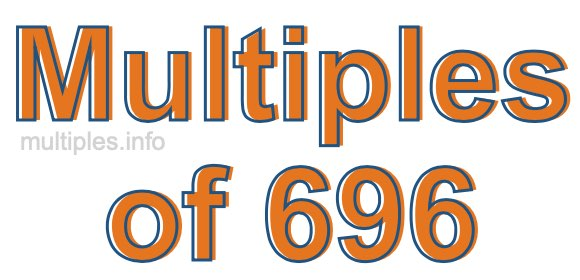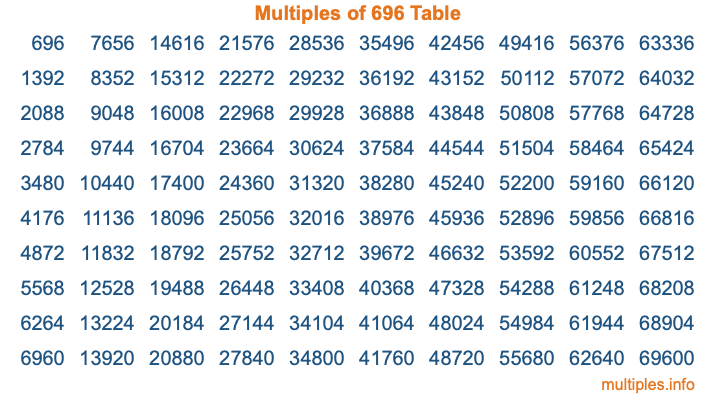Multiples of 696Welcome to the Multiples of 696 page. Here we will first teach you everything you will ever need to know about the multiples of 696, and then give you a study guide summary of everything we taught you to make sure you remember it all. Use this page to look up facts and learn information about the multiples of 696. This page will make you a multiples of six hundred ninety-six expert!

Definition of Multiples of 696
Multiples of 696 are all the numbers that when divided by 696 equal an integer. Each of the multiples of 696 are called a multiple. A multiple of 696 is created by multiplying 696 by an integer.

Therefore, to create a list of multiples of 696, you start with 1 multiplied by 696, then 2 multiplied by 696, then 3 multiplied by 696, and so on for as long as you want. Thus, the list of the first five multiples of 696 is 696, 1392, 2088, 2784, and 3480. To see a larger list of multiples of 696, see the printable image of Multiples of 696 further down on this page. We also have a category where you can choose any nth multiple of 696.

Multiples of 696 Checker
The Multiples of 696 Checker below checks to see if any number of your choice is a multiple of 696. In other words, it checks to see if there is any number (integer) that when multiplied by 696 will equal your number. To do that, we divide your number by 696. If the the quotient is an integer, then your number is a multiple of 696.

Is  a multiple of 696?

Least Common Multiple of 696 and ...
A Least Common Multiple (LCM) is the lowest multiple that two or more numbers have in common. This is also called the smallest common multiple or lowest common multiple and is useful to know when you are adding our subtracting fractions. Enter one or more numbers below (696 is already entered) to find the LCM.

Check out our LCM Calculator if you need more details about the Least Common Multiple or if you need the LCM for different numbers for adding and subtraction fractions.

nth Multiple of 696
As we stated above, 696 is the first multiple of 696, 1392 is the second multiple of 696, 2088 is the third multiple of 696, and so on. Enter a number below to find the nth multiple of 696.

th multiple of 696

Multiples of 696 vs Factors of 696
696 is a multiple of 696 and a factor of 696, but that is where the similarities end. All postive multiples of 696 are 696 or greater than 696. All positive factors of 696 are 696 or less than 696.

Below is the beginning list of multiples of 696 and the factors of 696 so you can compare:

Multiples of 696: 696, 1392, 2088, 2784, 3480, etc.

Factors of 696: 1, 2, 3, 4, 6, 8, 12, 24, 29, 58, 87, 116, 174, 232, 348, 696

As you can see, the multiples of 696 are all the numbers that you can divide by 696 to get a whole number. The factors of 696, on the other hand, are all the whole numbers that you can multiply by another whole number to get 696.

It's also interesting to note that if a number (x) is a factor of 696, then 696 will also be a multiple of that number (x).

Multiples of 696 vs Divisors of 696
The divisors of 696 are all the integers that 696 can be divided by evenly. Below is a list of the divisors of 696.

Divisors of 696: 1, 2, 3, 4, 6, 8, 12, 24, 29, 58, 87, 116, 174, 232, 348, 696

The interesting thing to note here is that if you take any multiple of 696 and divide it by a divisor of 696, you will see that the quotient is an integer.

Multiples of 696 Table
Below is an image of the first 100 multiples of 696 in a table. The table is in chronological order, column by column. The first column has the first ten multiples of 696, the second column has the next ten multiples of 696, and so on.The Multiples of 696 Table is also referred to as the 696 Times Table or Times Table of 696. You are welcome to print out our table for your studies.

Negative Multiples of 696
Although not often discussed or needed in math, it is worth mentioning that you can make a list of negative multiples of 696 by multiplying 696 by -1, then by -2, then by -3, and so on, to get the following list of negative multiples of 696:

-696, -1392, -2088, -2784, -3480, etc.

Multiples of 696 Summary
Below is a summary of important Multiples of 696 facts that we have discussed on this page. To retain the knowledge on this page, we recommend that you read through the summary and explain to yourself or a study partner why they hold true.

There are an infinite number of multiples of 696.

A multiple of 696 divided by 696 will equal a whole number.

696 divided by a factor of 696 equals a divisor of 696.

The nth multiple of 696 is n times 696.

The largest factor of 696 is equal to the first positive multiple of 696.

696 is a multiple of every factor of 696.

696 is a multiple of 696.

A multiple of 696 divided by a divisor of 696 equals an integer.

696 divided by a divisor of 696 equals a factor of 696.

Any integer times 696 will equal a multiple of 696.

Multiples of a Number
Here you can get the multiples of another number, all with the same attention to detail as we did for multiples of 696 on this page.

Multiples of
Multiples of 697
Did you find our page about multiples of six hundred ninety-six educational? Do you want more knowledge? Check out the multiples of the next number on our list!